Tuesday, April 18, 2006

Amelia_oh

 Today in first thing we did in Math is Mental Math. Write after that we were reviewing the polygon problem sheet from yesturday. Next Mr.Reece was showing us a new graph that has negative and positive numbers with a cross. Note from Mr. Reece. This graph is referred to as the coordinate plane. We use the coordinate plane for many different types of activities, from graphs, to maps, to the reproduction of objects. By using the coordinates we are able give precise directions and construct items with a great deal of precision.

Jeyson's Homework due April 19

Pattern #1: 2, 5, 8, 11, 14, 17, 20, 23
Start at 2 then keep on adding 3

Pattern #2: 99, 89, 79, 69, 59, 49
Start at 99 than keep on subtacting 10

Pattern #3: 1, 4, 9, 16, 19, 24, 31

Pattern#4: 1, 1, 2, 3, 5, 8, 13, 21, _, _, _
I don't know this one

Monday, April 17, 2006

April 12th, 13th, & 17th; Scribe

Here are somethings you need to know before reading this. When items are like this; (Note: Example), that means it's important. And when things are like this; (Example), it's just me blabbing. Bolded means you should either have them as notes, or try to remember them. You might need them on the exam. If the number 2 is bolded it means squared.

Wednesday April 12th

When we entered class we did our Mental Math as always. After reviewing those answers we learned the elements of a circle:
• Center; The middle of a circle
• Diameter; A line that goes from on edge of the other through the circle's center
• Radius; A line that travels from the center to the edge
• Circumference; Is the perimeter of a circle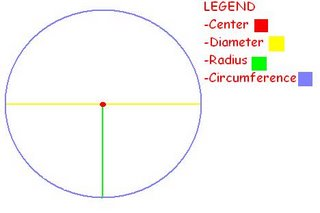We continued talking about the elements of a circle for the rest of the class.

Thursday April 13th
We did our Mental Math again as usual. We then had a meter stick, red string and two lids handed to our groups. We measured them on a table like this: (Note: "/" means divide.)(This is what my group did.)

Circle# Diameter Radius Circumference Circumference/ Diameter

9A 15CM 7CM 48CM 3.2CM
9B 29CM 11CM 70CM 2.41CM
7A 11CM 5CM 35CM 3.14CM
7B 14CM 7CM 43CM 3.07CM
4A 12CM 6CM 34CM 2.83CM
4B 29CM 11CM 70CM 2.41CM

After seeing the results of the circumference divided by the diameter, we noticed that they were close to 3.

Mr. Reece asked us what we thought the circumference divided by the diameter was. There were many guesses but one person said it:
Pi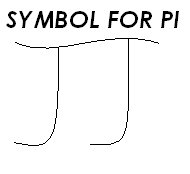Then we started talking about Pi and how it could help us find the circumference, diameter.

Here are the equations:
(Note: I'm only using the word because I can not put the symbol up. Usually you put the symbol instead of the word. "/" means divide, "X" means multiply.)

Diameter X Pi = Circumference

Circumference / Pi = Diameter

Radius X 2 X Pi = Circumference

After doing that we had a little pop quiz on Perimeter and Area.

For people who had missed Thursday and today:
HOMEWORK FROM THURSDAY:
Page 10.12 Entire page

Monday April 17th

We did mental math as usual, then we reviewed some questions some people had trouble on on the quiz.(That sounds right to me, if it is not please excuse me for my crappy english.)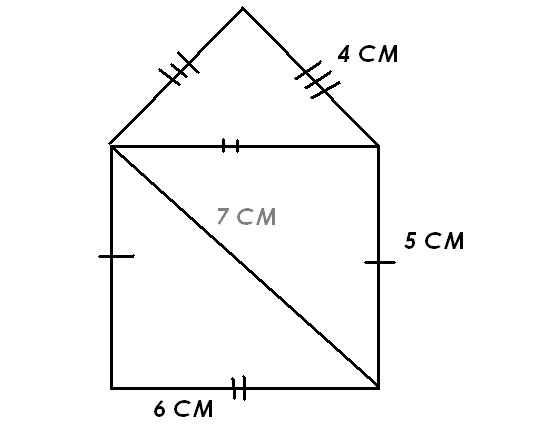This is one of the two problems people had trouble on. The question asked you to add the perimeter. Some people ended up adding the inside lines; 7 and 6. The proper answer would be 24.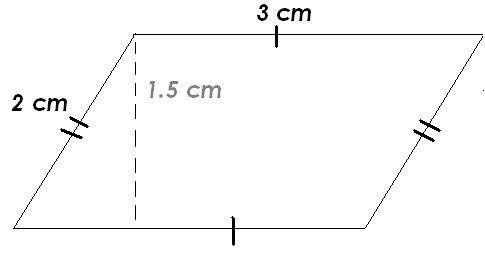Here's the second problem. Instead of multiplying 1.5 by 3, some people did 2 X 3. The proper answer is 4.5 CM2. Remember to add the 2 when you're talking about the area.

After that Mr. reece handed each of us a worksheet. The first three problems (A, B, C) where you had to find the area, and six more (D, E, F, G, H, I) where you had to determine the radius, diameter, circumference, and area, when rearranged(and also only showing the first letter) it spells out c.a.r.d (circumference, area, radius, diameter.)

To find out the area do Radius2 X Pi.

Here is A from the worksheet: (I tried my best to copy it, so don't bad mouth me.)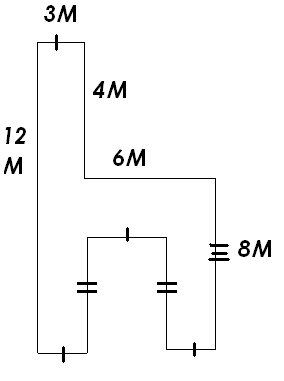Here is D from the worksheet: (Again, I tried my best to center everything.)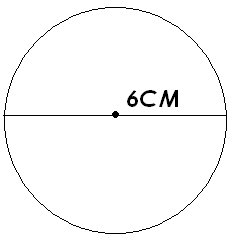For A try to find the area.(Pretty easy. W X H = A *wink wink*) For D try to find c.a.r.d. (If you don't know what c.a.r.d is look above.)

HOMEWORK FOR TONIGHT:
Finish worksheet handed back.

Sunday, April 16, 2006

Scribe List

I have decided to reset the scribe list. Everyone in the class who wishes to be the scribe for the day will earn the right to post the scribe notes. As well as the scribe posts are not mandatory, you can chose to opt out of the scribe posts. You must inform either myself or the previous scribe with your decision to not conplete the scribe post so that we can chose a new scribe. Remember you earn bonus marks upto the equivalent of two blogger assignments for completing the scribe post.

This is The Scribe List. Every possible scribe in our class is listed here. I will do my best to update this list every day. If you see someone's name crossed off on this list then you CANNOT choose them as the scribe for the next class.

This post is can be quickly accesed from the [Links] list over there on the right hand sidebar. Check here before you choose a scribe for tomorrow's class when it is your turn to do so.

Cycle 2